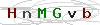UPSSS! JavaScript nie działa sprawdź ustawienia przeglądarkiInformacje o produkcie

## Selected problems in physics with examples and exercisesCena:

 Ilość35,00 zł

Dostępność: jest w magazynie sklepuDostępna ilość: 2
Najniższy koszt wysyłki to tylko 13,00 zł

Najedź aby zobaczyć wszystkie koszty wysyłki

 Specyfikacja książki Ilość stron 284 Okładka miękka Format B5 Rok wydania 2007 - wyd. II Język polski
Cena:

 Ilość35,00 zł

What is physics? The answer to this question has been changing, because physics also changes with time. But physics has always dealt with the basic rules governing the world: the macro-world as well as the world of atoms, electrons and nucleons. Physicists are concerned with various kinds of matter and radiation and their interactions. Their main purpose is to find, understand and use the basic laws that govern the natural world.
The foundation of physics is experiment. Experimental observation of the phenomena of nature allows physicists to find the rules and principles that relate these phenomena. This leads to formulation of models and theories, which form our perceptions of the investigated phenomena. Any physical model or theory must always be confronted with experiment. Essential contradictions between a particular model or theory and experimental data are a signal that the model or theory should be corrected or replaced by a new one. This confrontation is a source of development of physics and its continuous approaching a truth of nature.
Contemporary physics is a highly developed basic science with many fields, which are the foundation of all engineering and technology. Especially strong relations exist between physics and chemistry. Ever since chemistry ceased to be a purely phenomenological science, chemists not only employ certain laws of physics, but even include some complex and subtle physical phenomena and physics' arcane methods of measurement in their own research. This results in a gradual obliteration of the border line between some branches of chemistry and physics.

Spis treści:

1. INTRODUCTION

1.1. The nature of physics
1.2. The language and units. of physics
1.3. How to study physics?
Questions and problems

2. FUNDAMENTAL LAWS AND LAWS OF CONSERVATION IN PHYSICS

2.1. The meaning of fundamental laws
2.2. On the laws of conservation in physics
2.2.1. Newton's laws of motion
2.2.2. Conservation of momentum
2.2.3. Conservation of angular momentum
2.3. Work and energy
2.3.1. Definition of work in physics
2.3.2. Energy
2.3.3. The law of conservation of energy
2..3.3.1. Conservation of mechanical energy
2.3.3.2. Conservation of total energy
Questions and problems

3. KINETIC THEORY AND LAWS OF THERMODYNAMICS

3.1. The microscopic interpretation of temperature
3.2. Measuring temperature
3.3. Internal energy and equipartition of energy
3.4. Laws of thermodynamics
3.4.1. The first law of thermodynamics
3.4.2. The second law of thermodynamics
Questions and problems

4. ELECTROMAGNETIC INTERACTIONS

4.1. Static electric force
4.1.1. Electric charges
4.1.2. Quantization and conservation of charge
4.1.3. Electrical interaction between two charged particles - Coulomb's law
4.1.4. Electrical interactions in a system of several charges
4.2. Electric field
4.2.1. Graphic representation of electric fields
4.3. Gauss's law
4.3.1. Flux of electric field
4.3.2. Gauss's law
4.4. Electric potential and voltage
4.4.1. Electric potential energy
4.4.2. Electric potential
4.5. Capacitance and electric energy storage
4.5.1. Capacitors and capacitance
4.5.2. Energy storage
4.6. Electric current and magnetic force
4.6.1. Electric current
4.6.2. Ohm's law
4.6.3. Magnetic force and magnetic field
4.6.4. Magnetic field of linear current. Ampere's law
4.6.5. Gauss's law for magnetic field
4.7. Electromagnetic induction
4.7.2. Self-induced emf (?s
4.8. Maxwell's equations
Questions and problems

5. SELECTED PROBLEMS OF MODERN PHYSICS

5.1. The photoelectric effect
5.1.1. Photoelectrons
5.1.2. Fundamental features of the photoelectric effect
5.1.3. Einstein's theory of the photoelectric effect
5.2. Photons and electrons
5.2.1. X-ray photons
5.2.2. The Compton effect
5.3. The Bohr model of the atom of hydrogen
5.3. 1. Bohr's assumptions and postulates
5.3.2. Quantization of electron's total energy5.3.3. Electron jumps
5.3.4. Advantages and limitations of the Bohr model
5.4. Wave-particle duality
5.4.1. De Broglie's hypothesis
5.4.2. Experimental verification of de Broglie's hypothesis
Questions and problems

6. NUCLEAR PHYSICS

6.1. Nuclear size and structure
6.1.1. Constituents of the nucleus
6.1.2. Nuclear size
6.1.3. Nuclear forces
6.2.2. Beta decay
6.2.3. Alpha decay
6.2.4. Gamma decay
6.3. Nuclear reactions
6.3.1. General remarks
6.3.2. The fission reaction
6.3.3. Nuclear fusion
Questions and problems

EXERCISES
APPENDIX A. Mathematics

A.1. Vector algebra
A.2. Derivatives
A.3. Integrals

APPENDIX B.
ENGLISH-POLISH PHYSICS DICTIONARY.

Cena:

 Ilość35,00 zł

 Zapytaj o szczegóły
 Imię i nazwisko: E-mail:
Twoje pytanie:
Przepisz kod:Księgarnia Techniczna zamieszcza w ofercie głównie podręczniki akademickie oraz książki techniczne przede wszystkim z dziedzin takich jak mechanika techniczna, podstawy konstrukcji, technologia gastronomiczna. Główne wydawnictwa w ofercie to Politechnika Warszawska, Politechnika Wrocławska, Politechnika Świętokrzyska oraz POLSLKsięgarnia Techniczna - XML Sitemap